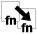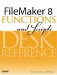# Number Calculations

#### fnHypotenuse ( leg1Length; leg2Length )

Although FileMaker includes many common mathematical operations and formulas, no list can be exhaustive. This function applies the Pythagorean Theorem (a2 + b2 = c2) to generate the length of a hypotenuse (the leg of a right triangle opposite the right angle).

Example:

fnHypotenuse ( 3; 4 ) returns 5.

Code:

``` // returns the length of a hypotenuse based on the Pythagorean
Theorem ( a^2 + b%2 = c%2 )
// input: two numbers
// output: number

Let ([
a2 = leg1Length * leg1Length;
b2 = leg2Length * leg2Length;
c2 = a2 + b2
];
Sqrt ( c2 )
)
```

#### fnNthRoot ( number; root )

FileMaker provides a built-in function for calculating the square root of a number, but not the nth root.

Example:

fnNthRoot ( 8; 3 ) returns 2.

fnNthRoot ( 256; 4 ) returns 4.

Code:

``` // returns the nth root of number
// input: two numbers
// output: number
Exp ( Ln ( number ) / root )
```This function computes the area of a regular polygon, given the number of sides and the radius of the polygon. (A regular polygon is a polygon in which all sides are of equal length.) The radius is the distance from the center of the polygon to any vertex. In other words, the radius of the polygon is the radius of a circle that exactly circumscribes the polygon.

Examples:

A pentagon with a radius of three meters would be evaluated like so: fnPolyAreaBySide ( 5 ; 3 ) which returns 21.399 (rounded) square meters.

An equilateral triangle with a radius of 4 inches: fnPolyAreaByRadius ( 3 ; 4 ) returns 20.723 square inches (rounded).

Code:

``` // computes the area of a regular polygon
// input:
// numberOfSides = the number of the polygons sides
// radius = distance from the center of the polygon to a vertex
// output: area of the polygon in aquare units
// requires fnPolyAreaFromSideLength

Let ([
n = numberOfSides;
sideLength = 2 * r * Sin ( Pi/n );
result = fnPolyAreaFromSideLength ( n ; sideLength )
];
result
)
```

#### fnPolyAreaFromSideLength( numberOfSides; sideLength )

This function computes the area of a regular polygon, given the number of sides and the length of each side.

Examples:

A hexagon with sides of length 3: fnPolyAreaBySide ( 6 ; 3 ) returns 23.382 (rounded) units squared.

An equilateral triangle with sides of length 4: fnPolyAreaBySide ( 3 ; 4 ) returns 6.928 (rounded) units squared.

Code:

``` // computes the area of a regular polygon
// input:
// numberOfSides = the number of the polygons sides
// sideLength = the length of one side
// output: area of the polygon in units squared

Let ([
n = numberOfSides;
l = sideLength;
result = ( n * l^2) / ( 4 * Tan( Pi/n ) )
];
result
)
```

#### fnRandomInRange ( lowNumber; highNumber )

The Random function in FileMaker returns a value from 0 to 1, but developers almost always need a random number within a range of numbers. For example, if you need a number between 10 and 50, the formula would be

``` Int ( Random * 41 ) + 10
```

This makes code somewhat difficult to read and requires that you think through the formula each time you need it. The fnRandomInRange() function hides this logic in an easy-to-use function.

Example:

fnRandomInRange ( 3; 7 ) might return 4.

Code:

``` // returns a random number from low to high range
// input: two numbers
// output: a random number within the range between the two
Int ( Random * ( highNumber - lowNumber + 1 )) + lowNumber
```

#### fnSphericalDistance ( lat1; long1; lat2; long2; units )

This function computes the distance between two points on the surface of the Earth, given in terms of decimal latitude and longitude. The coordinates must be decimalin other words, 45.5, not 45 degrees 30 minutesand must be given in degrees.

The function can return results in miles or kilometers. Any "units" value beginning with "m" will yield miles; otherwise, the function will return kilometers.

The computation is based on the "haversine formula" and assumes a reasonable degree of mathematical precision in the software, which FileMaker possesses.

See http://en.wikipedia.org/wiki/Haversine_formula for further details.

Example:

The distance between San Francisco and Chicago in miles is fnSphericalDistance ( 37.799; 122.461; 41.886; 87.623; "miles" ), which returns 1856.62.

Code:

``` // computes distance between two points on Earths surface
// input:
// lat1, long2, lat2, long2 = lat and long of two points, in DECIMAL DEGREES
// units = "miles" or "km"
// output: distance between the two points in miles or kilometers

Let([
D = Case( Trim(Lower(Left(units;1))) = "m"; 3958.75;6367.45 );
// diameter of Earth in miles or km
dlat = lat2R - lat1R;
dlong = long2R - long1R;
a = (Sin(dlat/2))^2 + Cos(lat1R) * Cos(lat2R) * (Sin(dlong/2))^2;
c = 2 * Atan(Sqrt(a)/Sqrt(1-a));
result = D * c
];
result
)
```FileMaker 8 Functions and Scripts Desk Reference
ISBN: 0789735113
EAN: 2147483647
Year: 2004
Pages: 352